﻿ 用Python估计离散选择模型 Estimate Discrete Choice Models Using Python – 王灿的个人网站

## 用Python估计离散选择模型 Estimate Discrete Choice Models Using Python

pylogit包的官方资源网站：https://github.com/timothyb0912/pylogit

pylogit_nlogit_commands.zip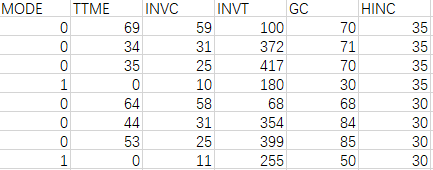MODE是因变量，TTME、INVC、INVT、HINC是自变量。其中，TTME、INVC、INVT是描述备选项的，一次选择中，不同选项的取值不同；而HINC是描述选择者的个体属性的，自然不会因为备选项的不同而不同。由于选择模型是基于效用差异的（only differences in utility matter），所以对于前者(TTME、INVC、INVT)，既然每个备选项的取值已经有了差异，就可以用一个通用的系数，被称为generic coefficients；而对于后者(HINC)，由于各备选项取值是一样的，没有产生差异，那么就必须在系数上产生差异——除了一个对照选项外，每一个选项都对应一个系数，被称为alternative-specific coefficients，特别地，常数项也属于此类变量，被称为alternative-specific constants (ASC)。

# MNL模型-1：只包含备选项的属性（generic coefficients）

VAir = βTTME * TTMEAir + βINVC * INVCAir + βINVT * INVTAir
VTrain = βTTME * TTMETrain + βINVC * INVCTrain + βINVT * INVTTrain
VBus = βTTME * TTMEBus + βINVC * INVCBus + βINVT * INVTBus
VCar = βTTME * TTMECar + βINVC * INVCCar + βINVT * INVTCar

NLOGIT通过以下程序估计这个模型：

`nlogit`
` ;lhs = mode`
` ;choices = air, train, bus, car`
` ;rhs = ttme,invc,invt`
` \$`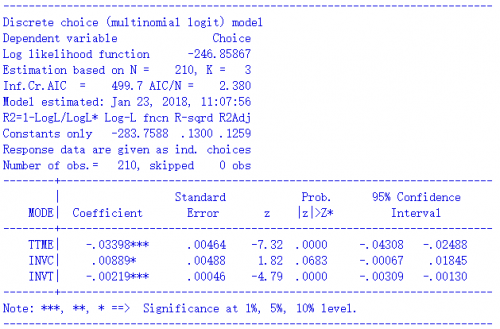`import pandas as pd`
` data = pd.read_csv("D:/TrafficModeData.csv")`
` data["Group"] = repmat(np.array([x+1 for x in range(210)]).reshape(210,1),1,4).reshape(840,1)`
` data["ALT"] = repmat(np.array([1,2,3,4]).reshape(4,1),210,1)`
` print(data.head(8))`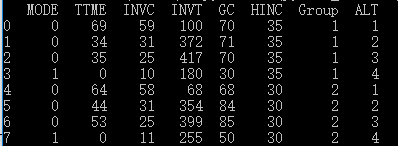`from collections import OrderedDict`
`spec = OrderedDict() variable_names = OrderedDict()`
` spec["TTME"] = [ [1, 2, 3, 4] ] variable_names["TTME"] = ["beta of TTME"] `

`Vars = ["TTME", "INVC", "INVT"]`
` for var in Vars:`
`   spec[var] = [[1,2,3,4]]`
`   variable_names[var] = [var] variable_names[var] = [var]`

`import pylogit as pl`
`model = pl.create_choice_model(data = data,`
`                    alt_id_col="ALT",`
`                    obs_id_col="Group",`
`                    choice_col="MODE",`
`                    specification=spec,`
`                    model_type = "MNL",`
`                    names = variable_names`
` )`

`model.fit_mle(np.zeros(3))`

`ValueError: The initial values are of the wrong dimension.It should be of dimension 3. But instead it has dimension 8`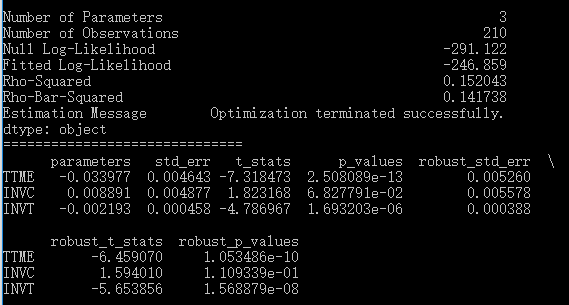# MNL模型-2：包含选择者的个体特征（alternative-specific coefficients）

VAir = βTTME * TTMEAir + βINVC * INVCAir + βINVT * INVTAir  + βAir + βAir_HINC * HINC
VTrain = βTTME * TTMETrain + βINVC * INVCTrain + βINVT * INVTTrain + βTrain + βTrain_HINC * HINC
VBus = βTTME * TTMEBus + βINVC * INVCBus + βINVT * INVTBus + βBus + βBus_HINC * HINC
VCar = βTTME * TTMECar + βINVC * INVCCar + βINVT * INVTCar

`nlogit`
`;lhs = mode`
`;choices = air, train, bus, car`
`;rhs = ttme,invc,invt`
`;rh2 = one, hinc`
`\$`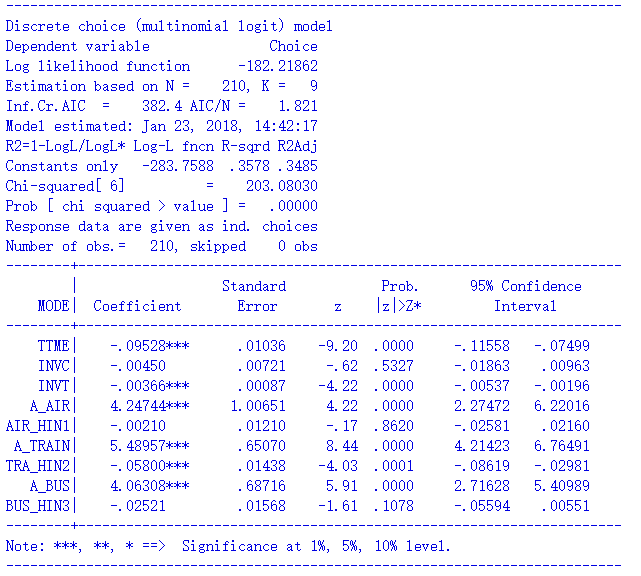`spec = OrderedDict()`` variable_names = OrderedDict()`` Vars = ["TTME", "INVC", "INVT"]`` for var in Vars:`
`   spec[var] = [[1,2,3,4]]`
`   variable_names[var] = [var]`` spec["intercept"] = [1,2,3]`` variable_names["intercept"] = ["ASC Air", "ASC Train", "ASC Bus"]`` spec["HINC"] = [1,2,3]`` variable_names["HINC"] = ["HINC for Air", "HINC for Train", "HINC for Bus"]`` model = pl.create_choice_model(data = data,`
`                    alt_id_col="ALT",`
`                    obs_id_col="Group",`
`                    choice_col="MODE",`
`                    specification=spec,`
`                    model_type = "MNL",`
`                    names = variable_names`` )`` model.fit_mle(np.zeros(9))`` model.print_summaries()model.print_summaries()`

# MNL模型-3：更灵活的设定

VAir = βTTME1 * TTMEAir + βINVC * INVCAir + βINVT * INVTAir
VTrain = βTTME2 * TTMETrain + βINVC * INVCTrain + βINVT * INVTTrain
VBus = βTTME2 * TTMEBus + βINVC * INVCBus + βINVT * INVTBus
VCar = βTTME2 * TTMECar + βINVC * INVCCar + βINVT * INVTCar  + βHINC * HINC

`nlogit`
`;lhs = mode;choices = air, train, bus, car`
`;model: U(air) = b_air + b_ttme1 * ttme + b_invc * invc + b_invt * invt/   `
`     U(train) = b_train + b_ttme2 * ttme + b_invc * invc + b_invt * invt/   `
`     U(bus) = b_bus + b_ttme2 * ttme + b_invc * invc + b_invt * invt/   `
`     U(car) = b_ttme2 * ttme + b_invc * invc + b_invt * invt + b_hinc * hinc`
`\$`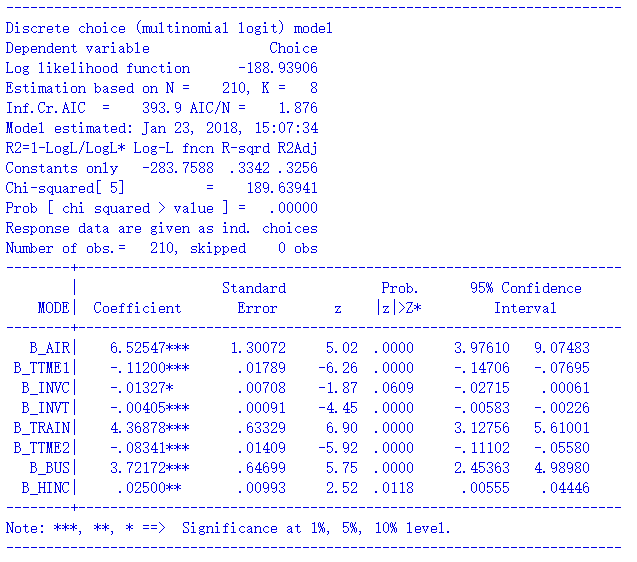`spec = OrderedDict()`` variable_names = OrderedDict()`` Vars = ["TTME", "INVC", "INVT"]`` spec["intercept"] = [1,2,3]`` variable_names["intercept"] = ["ASC Air", "ASC Train", "ASC Bus"]`` for var in Vars:`
`   spec[var] = [[1,2,3,4]]`
`   variable_names[var] = [var]`` spec["HINC"] = `` variable_names["HINC"] = ["HINC for Car"]`` spec["TTME"] = [, [2,3,4]]`` variable_names["TTME"] = ["TTME for Air", "TTME for Train/Bus/Car"]`` model = pl.create_choice_model(data = data,`
`                    alt_id_col="ALT",`
`                    obs_id_col="Group",`
`                    choice_col="MODE",`
`                    specification=spec,`
`                    model_type = "MNL",`
`                    names = variable_names`` )`` model.fit_mle(np.zeros(8))`` model.print_summaries()model.print_summaries()`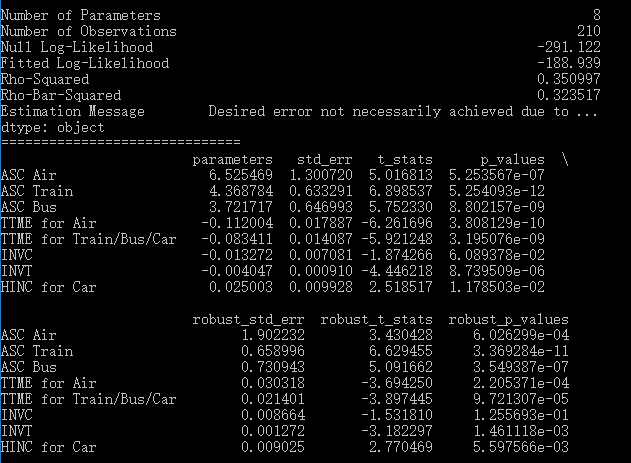# 估计完成的后续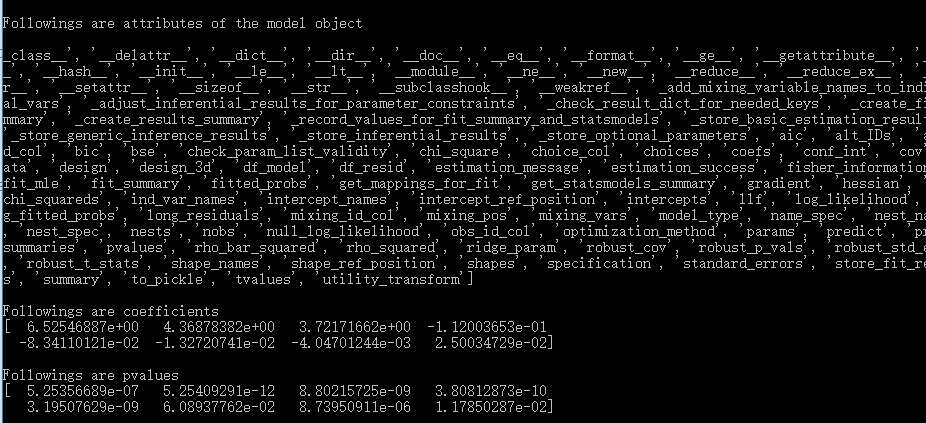`print("\n\nFollowing are choosing probabilities of all alternatives in the first 2 cases")`
`print(model.predict(data.iloc[0:8]))`
`print("\nFollowing are choosing probabilities of actually chosen alternatives in the first 2 cases")`
`print(model.predict(data.iloc[0:8],choice_col="MODE", return_long_probs=False))`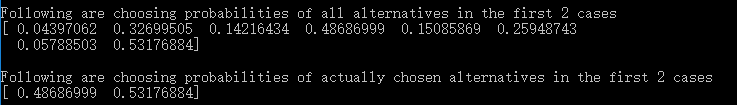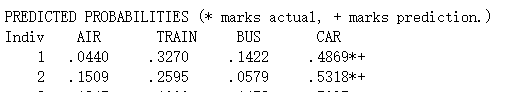# Nested Logit模型

`nest_membership = OrderedDict()`
`nest_membership["Fly"] = `
`nest_membership["Ground"] = [2,3,4]`

`model = pl.create_choice_model(data = data,`
`                    alt_id_col="ALT",`
`                    obs_id_col="Group",`
`                    choice_col="MODE",`
`                    specification=spec,`
`                    names = variable_names,`
`                    model_type = "Nested Logit",`
`                    nest_spec = nest_membership`
` )`

`model.fit_mle(np.zeros(11))`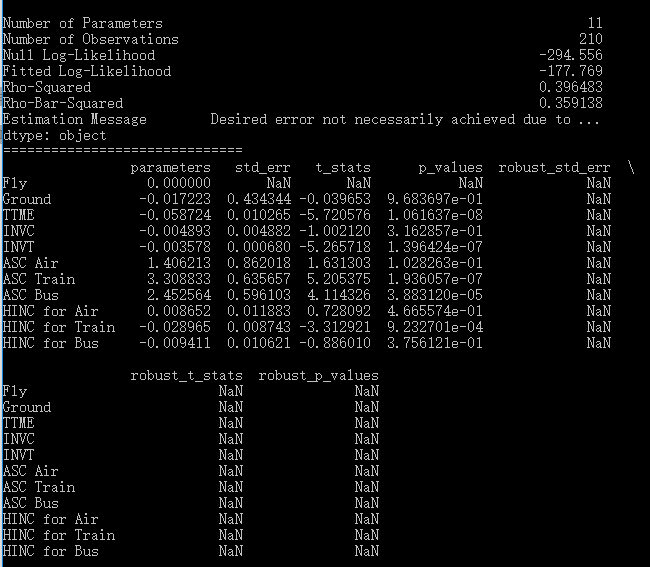`nlogit`
`;lhs = mode`
`;choices = air, train, bus, car`
`;rhs = ttme,invc,invt`
`;rh2 = one, hinc`
`;tree = travel[fly(air), group(train, bus, car)]`
`;ru2`
`\$`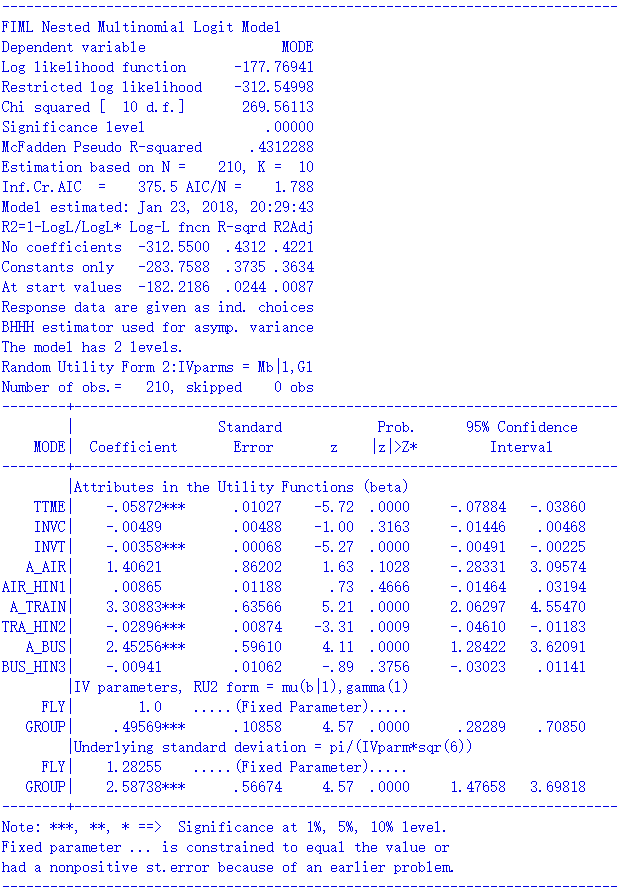`nest_membership["Private"] = [1,4]`
`nest_membership["Public"] = [2,3]`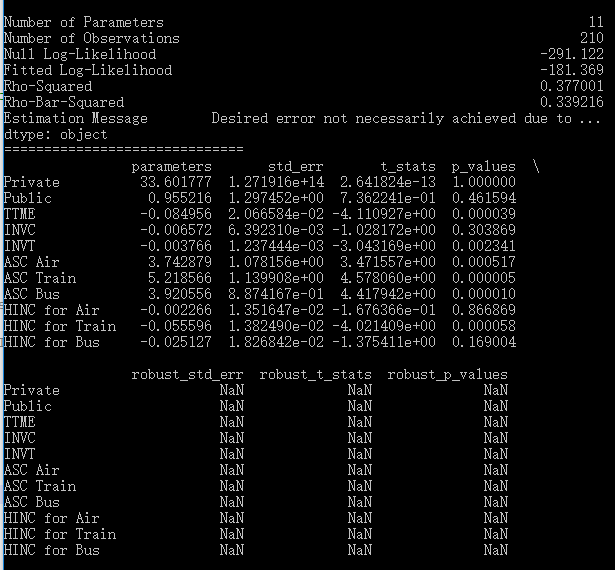;tree = travel[public(train, bus), private(air, car)]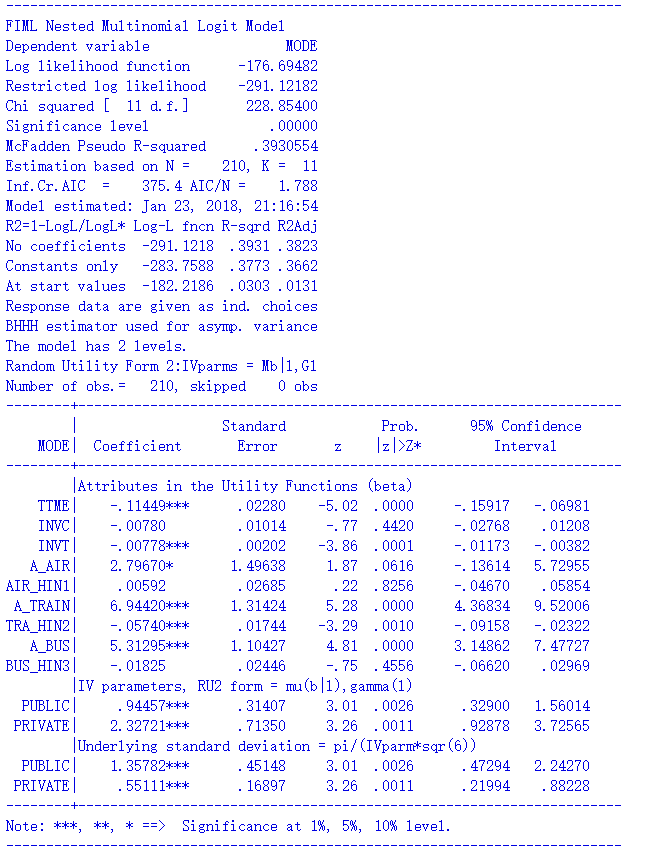`label def mode_name 1 "Air" 2 "Train" 3 "Bus" 4 "Car"`
`label values alt mode_name`
`nlogitgen type = alt(public: 2|3, private:1|4)`
`nlogittree alt type, choice(mode)`
`nlogit mode ttme invc invt || type: || alt: hinc, base(4) , case(group)`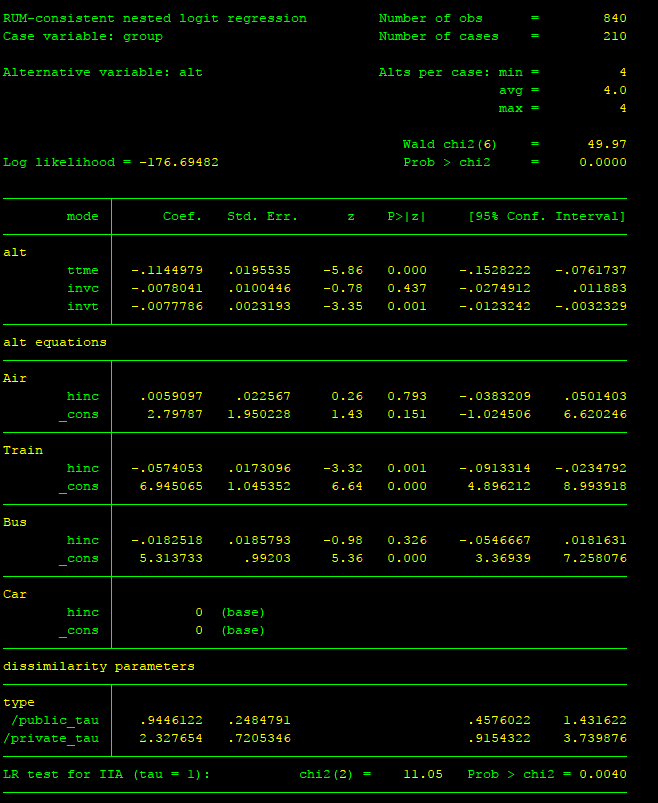# Mixed Logit模型

pylogit还可以提供了mixed logit模型的估计功能，为此需要在pl.create_choice_model中增加两个字段：其一是“mixing_vars”，以变量名列表的形式定义哪些变量是随机的，从官方的说明来看，似乎只支持正态分布假设；其二是“mixing_id_col”，用来定义这些变量在哪些样本上分布。

`spec = OrderedDict()`
`variable_names = OrderedDict()`
`Vars = ["TTME", "INVC", "INVT"]`
`for var in Vars:`
`   spec[var] = [[1,2,3,4]]`
`   variable_names[var] = [var]`
`spec["intercept"] = [1,2,3]`
`variable_names["intercept"] = ["ASC Air", "ASC Train", "ASC Bus"]`
`spec["HINC"] = [1,2,3]`
`variable_names["HINC"] = ["HINC for Air", "HINC for Train", "HINC for Bus"]`
`model = pl.create_choice_model(data = data,`
`                    alt_id_col="ALT",`
`                    obs_id_col="Group",`
`                    choice_col="MODE",`
`                    specification=spec,`
`                    names = variable_names,`
`                    model_type = "Mixed Logit",`
`                    mixing_id_col="Group",`
`                    mixing_vars = ["TTME", "INVC", "INVT"]`
` )`
`model.fit_mle(np.zeros(12), num_draws=1000)`
`model.print_summaries()`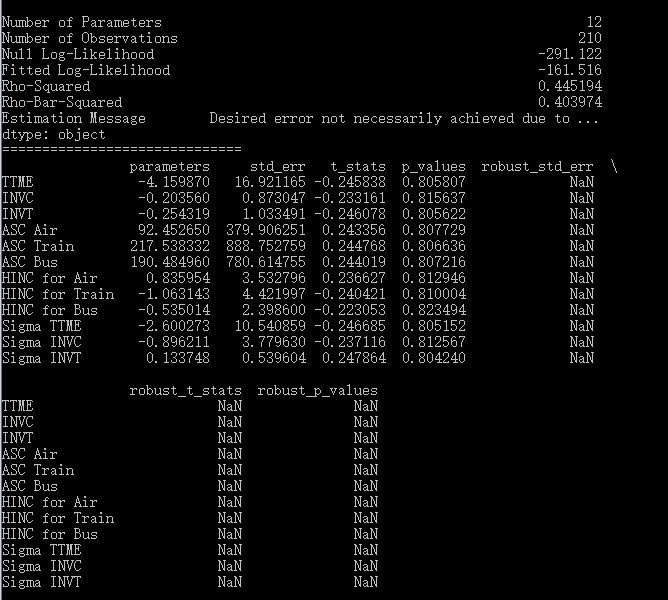`nlogit`
`;lhs = mode`
`;choices = air, train, bus, car`
`;rhs = ttme,invc,invt`
`;rh2 = one, hinc`
`;fcn = ttme(n), invc(n), invt(n)`
`;rpl`
`;pts = 1000`
`;halton`
`\$`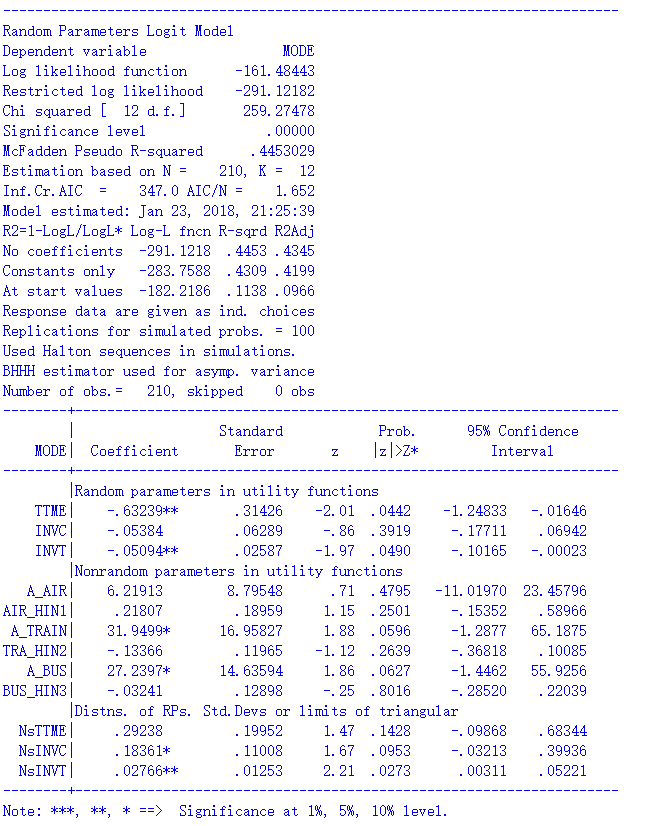### This Post Has 8 Comments

1.请问在stata中是不是无法在某个选择肢的效用函数中加入特有的变量？这个问题类似于本文章的MNL模型-3：更灵活的设定。

1.可以的。设有3个选项A/B/C，有一个变量x只适用于C，只需要把A/B在x上的取值设为0就可以了。

1.谢谢回答！我时隔近一年我才看到。我又遇到问题了。我想做混合选择模型就是（Mixed logit+latent variables）,我知道pandasbiogeme可以做，但是吧，它的例子数据没有我的复杂。我想问pandas这个包，怎么考虑不同sp中选择肢个数不同的情况？另外我的调查问卷进行了两次调查，第一次调查每份问卷4个场景，第二次调查每份问卷2个场景。这个问题NLOGIT软件中我找打了答案。我也想用NOLGOT做混合选择模型，但是参考手册里面没有提到，有一本资料有提到，但是描述得太少，不太明白。

1.我也用过biogeme，biogeme可以自己设计效应函数，我看这个跟xlogit是不是都不行

2.如果每个人的choice set 不同 有什么解决办法吗

1.Nlogit倒是可以设置，lhs中加入cset这个变量，这个变量得值就是每个sp中得选择肢个数

1.是的，其实我肯定是更推荐用NLogit的。这个应该是最权威的结果，而且我发现pylogit给出的mxl的结果与Nlogit的结果有相当多的不一致，即使考虑到simulation中的随机因素。

2.我也用过biogeme，biogeme可以自己设计效应函数，我看这个跟xlogit是不是都不行

ICP备案号：沪ICP备20010289号-1